• WIFI信号强度在-20dBM 至 -70 dBm之间，越小越好，超过-70dBm可以说信号差。 无线连接速率：2.4G单天线最大150M速率 5G 单天线最大433M速率 推荐连接5G方可获得更大的带宽速率。 无线频率：太专业术语自己百.
 火山安卓识别周边最佳手机WIFI环境(并判断是否支持5G)
经常听大家说手机网络怎么这么慢呢？有没有什么好办法判断下自己周边的网络环境究竟如何，并进行改善优化呢？答案是必须的，下面我们就用火山安卓实现一款简易的WIFI信息查看小工具。

额外福利，知识拓展：
WIFI信号强度在-20dBM 至 -70 dBm之间，值越小越好，超过-70dBm可以说信号极差。
无线连接速率：2.4G单天线最大150M速率  5G 单天线最大433M速率 推荐连接5G方可获得更大的带宽速率。
无线频率：太专业术语自己百度，只说简单可以理解的原则：在列表框搜索到的wifi信号找相同频率最少的WIFI去连接，干扰才最小。
好开始我们今天的课程。
第一步 打开火山安卓编程工具，新建项目。
   都会，图略

第二步   设计安卓APP界面（由于是DEMO，并未做美化）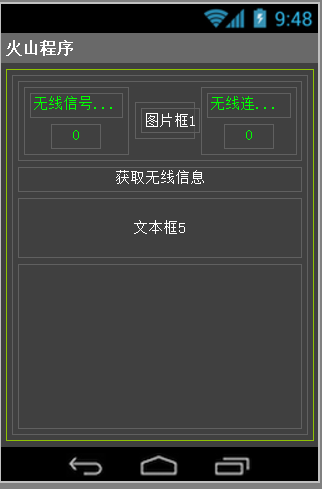第三步：编写代码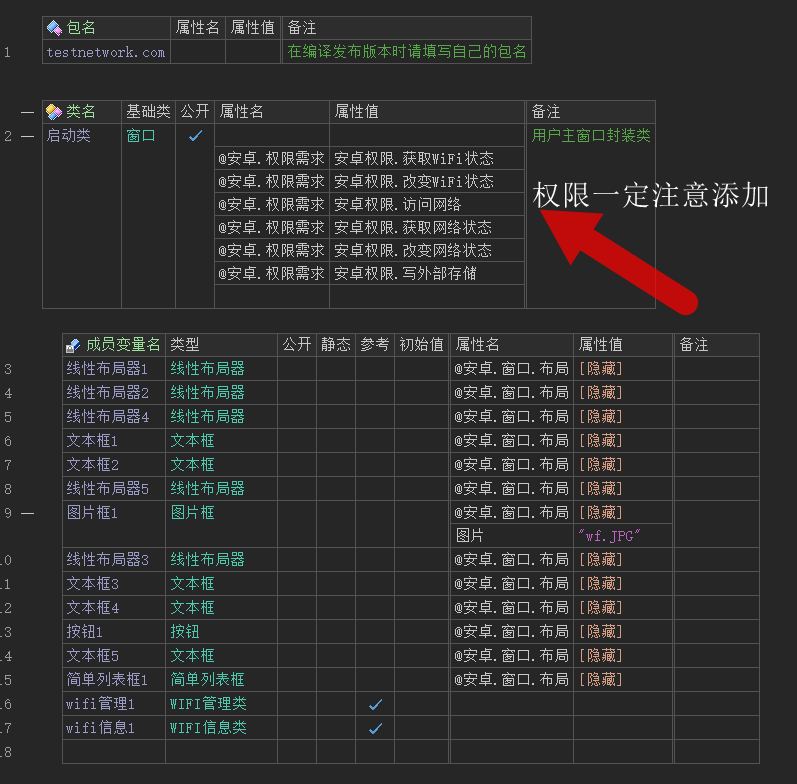注意：安卓在8.0及9.0后获取wifi的ssid需要添加GPS位置信息获取的权限
在窗口被创建时实例化WIFI管理类。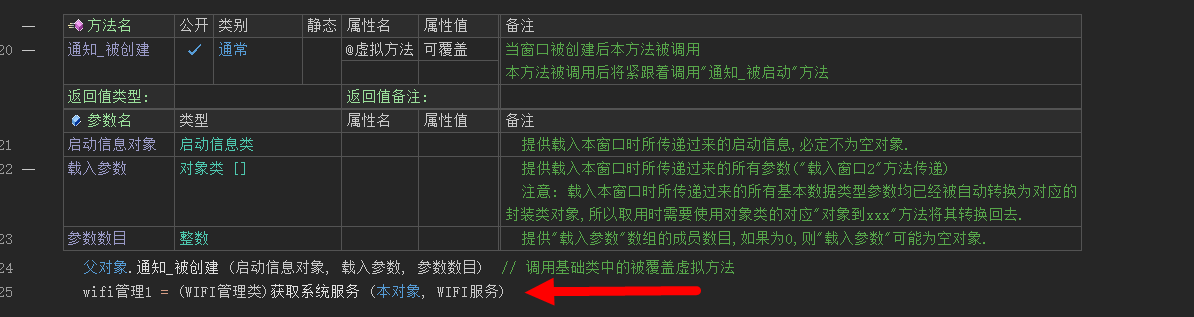编写按钮单击事件的代码：进行WIFI信息的获取和周围WIFI信号的扫描。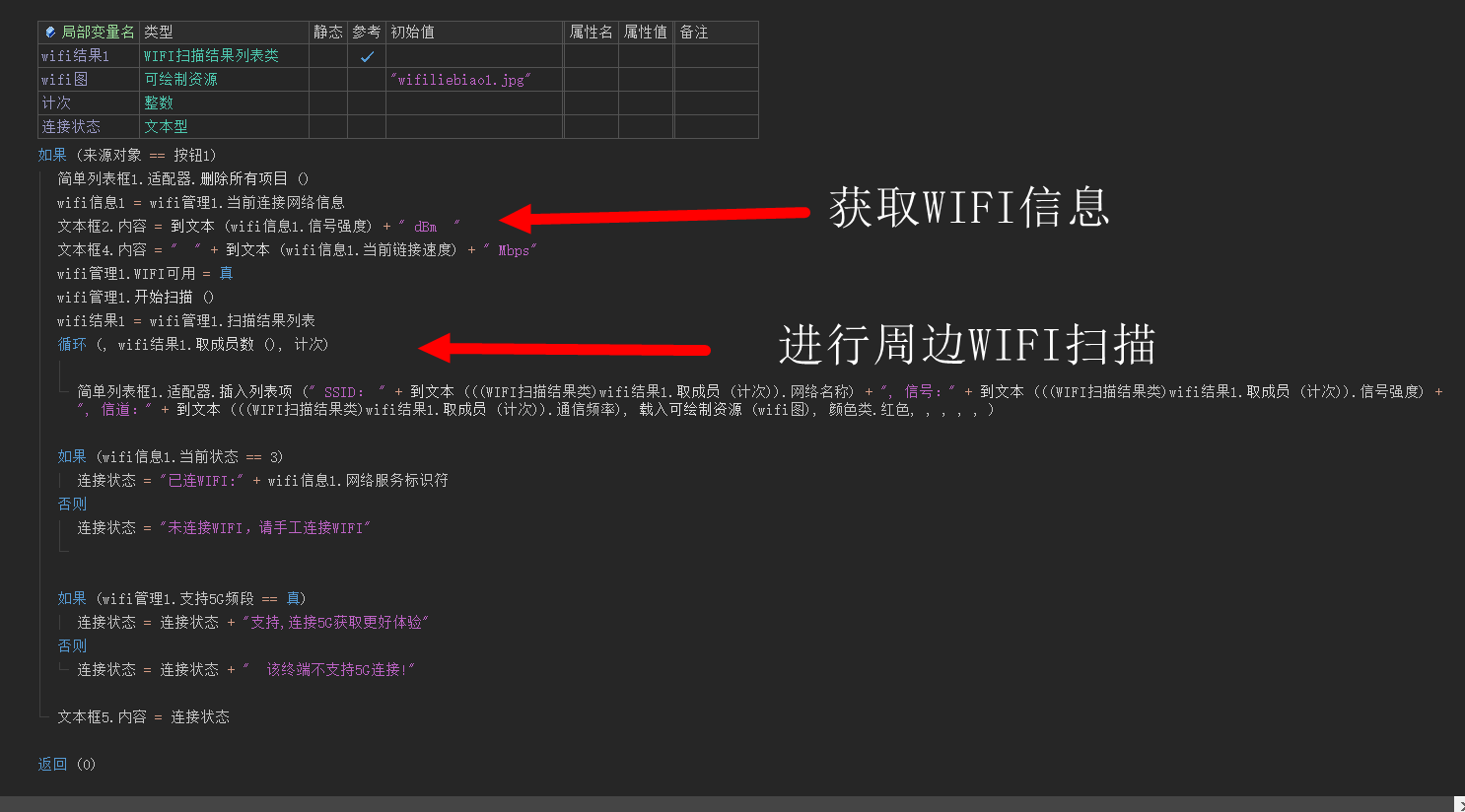下图是在模拟器运行的结果，真实环境会扫描到非常多的WIFI小伙伴们是不是超级简单？赶紧动手尝试一下，可以在这个基础上编写自己的WIFI分析仪
加油！
源码下载：点击本链接


展开全文c++ c语言
• 第二十八讲：正定矩阵和最小值本讲学习正定矩阵positive definite matrices，这个主题把整门课的知识融为一体，主元，行列式，特征值，不稳定性，新表达式xTAxx^TAx。...当极小值存在时，如何找出极小值
第二十八讲：正定矩阵和最小值

本讲学习正定矩阵positive definite matrices，这个主题把整门课的知识融为一体，主元，行列式，特征值，不稳定性，新表达式xTAx$x^TAx$。目标是：怎么判断一个矩阵是否是正定矩阵$\color{red}{怎么判断一个矩阵是否是正定矩阵}$，为什么对正定矩阵感兴趣，最后给出几何上的解释，椭圆和正定性有关，双曲线与正定性无关。当极小值存在时，如何找出极小值应用。本讲涉及的矩阵均为实对称矩阵。$\color{red}{本讲涉及的矩阵均为实对称矩阵。}$

1.正定矩阵

1.1正定性的判断

正定矩阵判定方法$\color{red}{正定矩阵判定方法}$：

1）特征值方法：λi>0$λ_i>0$；
2）行列式方法：所有顺序主子阵（leading principal submatrix）的行列式（即顺序主子式，leading principal minor）大于零；
3）主元方法：矩阵消元后主元均大于零；
4）新方法：xTAx>0$x^TAx>0$，x$x$ 是任意向量，除零向量外。
大多数情况下使用4)来定义正定性，而用前三条来验证正定性。

我们仍然从二阶说起，有矩阵A=[abbd]$A=\begin{bmatrix}a& b\\b& d\end{bmatrix}$，判断其正定性有以下方法：
1.矩阵的所有特征值大于零则矩阵正定：λ1>0,λ2>0$\lambda_1>0,\lambda_2>0$；
2.矩阵的所有顺序主子阵（leading principal submatrix）的行列式（即顺序主子式，leading principal minor）大于零则矩阵正定：a>0, ac−b2>0$a>0,\ ac-b^2>0$；
3.矩阵消元后主元均大于零：a>0, ac−b2a>0$a>0,\ \frac{ac-b^2}{a}>0$；
4.xTAx>0$x^TAx>0$；

大多数情况下使用4来定义正定性，而用前三条来验证正定性。

1.2 最小值的判定及其几何意义

双曲线、抛物线、椭圆之间的联系与区别：
联系：它们都属于圆锥曲线；
区别：根本的差别在于它们的离心率e不同，抛物线的离心率e=1为常数，双曲线的离心率e＞1，椭圆的离心率0＜e＜1。
e=a2+b2−−−−−−√$e=\sqrt{a^2+b^2}$，a是长轴的长度，b是短轴的长度。

例如：
来计算一个例子：A=[266?]$A=\begin{bmatrix}2& 6\\6& ?\end{bmatrix}$，在?$?$处填入多少才能使矩阵正定？

1）来试试18$18$，此时矩阵为A=$A=\begin{bmatrix}2& 6\\6& 18\end{bmatrix}$，detA=0$\det A=0$，此时的矩阵成为半正定矩阵（positive semi-definite）。矩阵奇异，其中一个特征值必为0$0$，从迹得知另一个特征值为20$20$。矩阵的主元只有一个，为2$2$。
计算xTAx$x^TAx$，得
[x1x2][x1x2]=2x21+12x1x2+18x22\begin{bmatrix}x_1& x_2\end{bmatrix}\begin{bmatrix}2& 6\\6& 18\end{bmatrix}\begin{bmatrix}x_1\\x_2\end{bmatrix}=2x_1^2+12x_1x_2+18x_2^2
这样我们得到了一个关于x1,x2$x_1,x_2$的函数f(x1,x2)=2x21+12x1x2+18x22$f(x_1,x_2)=2x_1^2+12x_1x_2+18x_2^2$，这个函数不再是线性的，在本例中这是一个纯二次型（quadratic）函数，它没有线性部分、一次部分或更高次部分（Ax$Ax$是线性的，但引入xT$x^T$后就成为了二次型）。
当?取18时，判定1、2、3都是 “刚好不及格”$\color{red}{“刚好不及格”}$。

半正定矩阵：不是正定矩阵，是对称的，是成为正定矩阵的临界点，奇异矩阵，有一个特征值为0，其他特征值大于等于0。$\color{red}{半正定矩阵：不是正定矩阵，是对称的，是成为正定矩阵的临界点，奇异矩阵，有一个特征值为0，其他特征 值大于等于0。}$

2)我们可以先看“一定不及格$\color{red}{一定不及格}$”的样子，令?=7$?=7$，矩阵为A=$A=\begin{bmatrix}2& 6\\6& 7\end{bmatrix}$，二阶顺序主子式变为−22$-22$，显然矩阵不是正定的，此时的函数为f(x1,x2)=2x21+12x1x2+7x22$f(x_1,x_2)=2x_1^2+12x_1x_2+7x_2^2$，如果取x1=1,x2=−1$x_1=1,x_2=-1$则有f(1,−1)=2−12+7<0$f(1,-1)=2-12+7<0$。

几何意义: 如果我们把z=2x2+12xy+7y2$z=2x^2+12xy+7y^2$放在直角坐标系中，图像过原点z(0,0)=0$z(0,0)=0$，当y=0$y=0$或x=0$x=0$或x=y$x=y$时函数为开口向上的抛物线，所以函数图像在某些方向上是正值；而在某些方向上是负值，比如x=−y$x=-y$，所以函数图像是一个马鞍面（saddle），(0,0,0)$(0,0,0)$点称为鞍点（saddle point），它在某些方向上是极大值点，而在另一些方向上是极小值点。（实际上函数图像的最佳观测方向是沿着特征向量的方向。）

3)再来看一下“一定及格$\color{red}{一定及格}$”的情形，令?=20$?=20$，矩阵为A=$A=\begin{bmatrix}2& 6\\6& 20\end{bmatrix}$，行列式为detA=4$\det A=4$，迹为trace(A)=22$trace(A)=22$，特征向量均大于零，矩阵可以通过测试。此时的函数为f(x1,x2)=2x21+12x1x2+20x22$f(x_1,x_2)=2x_1^2+12x_1x_2+20x_2^2$，函数在除(0,0)$(0,0)$外处处为正。

几何意义:我们来看看z=2x2+12xy+20y2$z=2x^2+12xy+20y^2$的图像，式子的平方项均非负，所以需要两个平方项之和大于中间项即可，该函数的图像为抛物面（paraboloid）。在(0,0)$(0,0)$点函数的一阶偏导数均为零，二阶偏导数均为正（马鞍面的一阶偏导数也为零，但二阶偏导数并不均为正，所以），函数在该点取极小值。

在微积分中，一元函数取极小值需要:

一阶导数为零且二阶导数为正dudx=0,d2udx2>0$\frac{\mathrm{d}u}{\mathrm{d}x}=0, \frac{\mathrm{d}^2u}{\mathrm{d}x^2}>0$。
在线性代数中我们遇到了了多元函数f(x1,x2,⋯,xn)$f(x_1,x_2,\cdots,x_n)$，要取极小值需要二阶偏导数矩阵为正定矩阵。

在本例中（即二阶情形），如果能用平方和的形式来表示函数，则很容易看出函数是否恒为正，f(x,y)=2x2+12xy+20y2=2(x+3y)2+2y2$f(x,y)=2x^2+12xy+20y^2=2\left(x+3y\right)^2+2y^2$。另外，如果是上面的?=7$?=7$的情形，则有f(x,y)=2(x+3y)2−11y2$f(x,y)=2(x+3y)^2-11y^2$，如果是?=18$?=18$的情形，则有f(x,y)=2(x+3y)2$f(x,y)=2(x+3y)^2$。

如果令z=1$z=1$，相当于使用z=1$z=1$平面截取该函数图像，将得到一个椭圆曲线。另外，如果在?=7$?=7$的马鞍面上截取曲线将得到一对双曲线。

再来看这个矩阵的消元，=[1−301]$\begin{bmatrix}2& 6\\6& 20\end{bmatrix}=\begin{bmatrix}1& 0\\-3& 1\end{bmatrix}\begin{bmatrix}2& 6\\0& 2\end{bmatrix}$，这就是A=LU$A=LU$，可以发现矩阵L$L$中的项与配平方中未知数的系数有关，而主元则与两个平方项外的系数有关，这也就是为什么正数主元得到正定矩阵。

上面又提到二阶导数矩阵，对于二元函数取极小值需要（与一元函数类似）:

一阶偏导为0；
对于二阶导数，这个矩阵型为[fxxfyxfxyfyy]$\begin{bmatrix}f_{xx}& f_{xy}\\f_{yx}&f_{yy}\end{bmatrix}$，显然，矩阵中的主对角线元素（纯二阶导数）必须为正，并且主对角线元素必须足够大来抵消混合导数的影响。同时还可以看出，因为二阶导数的求导次序并不影响结果，所以矩阵必须是对称的。

以此类推，现在我们就可以计算n×n$n\times n$阶矩阵了。

1.3 正定矩阵的拓展

接下来计算一个三阶矩阵，A=⎡⎣⎢2−10−12−10−12⎤⎦⎥$A=\begin{bmatrix}2& -1& 0\\-1& 2& -1\\0& -1& 2\end{bmatrix}$，它是正定的吗？函数xTAx$x^TAx$是多少？函数在原点去最小值吗？图像是什么样的？

先来计算矩阵的顺序主子式，分别为2,3,4$2,3,4$；再来计算主元，分别为2,32,43$2,\frac{3}{2},\frac{4}{3}$；计算特征值，λ1=2−2√,λ2=2,λ3=2+2√$\lambda_1=2-\sqrt 2,\lambda_2=2,\lambda_3=2+\sqrt 2$。(正定)
计算xTAx=2x21+2x22+2x23−2x1x2−2x2x3$x^TAx=2x_1^2+2x_2^2+2x_3^2-2x_1x_2-2x_2x_3$。
图像是四维的抛物面，当我们在f(x1,x2,x3)=1$f(x_1,x_2,x_3)=1$处截取该面，将得到一个椭圆体。得到的图形则是一个扁的橄榄球，有一个长轴，另外两个轴相等，类似于一个矩阵有一重复的特征值，另一个不同（3 个特征值）。如果是球的话，那就是单位矩阵，所有的特征值相同。

一般的情况下，三个特征值都不相同，它相当于有一个长轴，一个中轴，一个短轴，三个轴的方向就是特征向量的方向，轴的长度由特征值大小来决定。$\color{red}{一般的情况下，三个特征值都不相同，它相当于有一个长轴，一个中轴，一个短轴，三个轴的方向就是特征向量的方向，轴的长度由特征值大小来决定。}$

我们将矩阵A$A$(对称矩阵)分解为A=QΛQT$A=Q\Lambda Q^T$，可以发现上面说到的各种元素都可以表示在这个分解的矩阵中，我们称之为主轴定理$\color{red}{主轴定理}$（principal axis theorem），即特征向量说明主轴的方向、特征值说明主轴的长度。

A=QΛQT$A=Q\Lambda Q^T$是特征值相关章节中最重要的公式。

2. 本章总结

正定矩阵判定方法$\color{red}{正定矩阵判定方法}$：

1）特征值方法：λi>0$λ_i>0$；
2）行列式方法：所有顺序主子阵（leading principal submatrix）的行列式（即顺序主子式，leading principal minor）大于零；
3）主元方法：矩阵消元后主元均大于零；
4）新方法：xTAx>0$x^TAx>0$，x$x$ 是任意向量，除零向量外。
大多数情况下使用4)来定义正定性，而用前三条来验证正定性。

最小值的判定：一阶偏导为0；二阶偏导大于0。
主轴定理：
将矩阵A$A$(对称矩阵)分解为A=QΛQT$A=Q\Lambda Q^T$，可以发现上面说到的各种元素都可以表示在这个分解的矩阵中，我们称之为主轴定理$\color{red}{主轴定理}$（principal axis theorem），即特征向量说明主轴的方向、特征值说明主轴的长度。

第二十九讲：相似矩阵和若尔当形

在本讲的开始，先接着上一讲来继续说一说正定矩阵。

正定矩阵的逆矩阵有什么性质？

我们将正定矩阵分解为A=SΛS−1$A=S\Lambda S^{-1}$，引入其逆矩阵A−1=SΛ−1S−1$A^{-1}=S\Lambda^{-1}S^{-1}$，我们知道正定矩阵的特征值均为正值，所以其逆矩阵的特征值也必为正值（即原矩阵特征值的倒数）所以，正定矩阵的逆矩阵也是正定的。
如果A, B$A,\ B$均为正定矩阵，那么A+B$A+B$呢？
我们可以从判定xT(A+B)x$x^T(A+B)x$入手，根据条件有xTAx>0, xTBx>0$x^TAx>0,\ x^TBx>0$，将两式相加即得到xT(A+B)x>0$x^T(A+B)x>0$。所以正定矩阵之和也是正定矩阵。
再来看有m×n$m\times n$矩阵A$A$，则ATA$A^TA$(最早出现在最小二乘法)具有什么性质？
我们在投影部分经常使用ATA$A^TA$，这个运算会得到一个对称矩阵，这个形式的运算用数字打比方就像是一个平方，用向量打比方就像是向量的长度平方，而对于矩阵，有ATA$A^TA$正定：在式子两边分别乘向量及其转置得到xTATAx$x^TA^TAx$，分组得到(Ax)T(Ax)$(Ax)^T(Ax)$，相当于得到了向量Ax$Ax$的长度平方，则|Ax|2≥0$|Ax|^2\geq0$。要保证模不为零，则需要Ax$Ax$的零空间中仅有零向量，即A$A$的各列线性无关（rank(A)=n$rank(A)=n$）即可保证|Ax|2>0$|Ax|^2>0$，ATA$A^TA$正定。
另外，在矩阵数值计算中，正定矩阵消元不需要进行“行交换”操作，也不必担心主元过小或为零，正定矩阵具有良好的计算性质。

接下来进入本讲的正题。

1. 相似矩阵

先列出定义：

矩阵A, B$A,\ B$对于某矩阵M$M$(可逆)满足B=M−1AM$B=M^{-1}AM$时，称A, B$A,\ B$互为相似矩阵。

1.对于在对角化一讲（第二十二讲）中学过的式子S−1AS=Λ$S^{-1}AS=\Lambda$（S$S$是特征向量组成的矩阵），则有A$A$相似于Λ$\Lambda$。

矩阵A$A$ 的所有相似矩阵里面，Λ$Λ$是最好的，还有许多其他矩阵与A 相似。我们可以用任意的可逆矩阵M 代替S,都得到一个新的矩阵，这个新的矩阵与A 相似。那么A 与其他所有的相似矩阵的共同点是什么？

1.1两大性质

性质：1）相似矩阵具有相同的特征值；（注意特征向量并不相同）$\color{red}{性质：1）相似矩阵具有相同的特征值；（注意特征向量并不相同）}$

举个例子，A=$A=\begin{bmatrix}2& 1\\1& 2\end{bmatrix}$，容易通过其特征值得到相应的对角矩阵Λ=$\Lambda=\begin{bmatrix}3& 0\\0& 1\end{bmatrix}$，取M=$M=\begin{bmatrix}1& 4\\0& 1\end{bmatrix}$，则B=M−1AM=[10−41]=[−21−156]$B=M^{-1}AM=\begin{bmatrix}1& -4\\0& 1\end{bmatrix}\begin{bmatrix}2& 1\\1& 2\end{bmatrix}\begin{bmatrix}1& 4\\0& 1\end{bmatrix}=\begin{bmatrix}-2& -15\\1& 6\end{bmatrix}$。

我们来计算这几个矩阵的的特征值（利用迹与行列式的性质），λΛ=3, 1$\lambda_{\Lambda}=3,\ 1$、λA=3, 1$\lambda_A=3,\ 1$、λB=3, 1$\lambda_B=3,\ 1$。 所以，相似矩阵有相同的特征值。

继续上面的例子，特征值为3, 1$3,\ 1$的这一族矩阵都是相似矩阵，如$\begin{bmatrix}3& 7\\0& 1\end{bmatrix}$、$\begin{bmatrix}1& 7\\0& 3\end{bmatrix}$，其中最特殊的就是Λ$\Lambda$。

证明：
现在我们来证明这个性质，有Ax=λx, B=M−1AM$Ax=\lambda x,\ B=M^{-1}AM$，第一个式子化为AMM−1x=λx$AMM^{-1}x=\lambda x$，接着两边同时左乘M−1$M^{-1}$得M−1AMM−1x=λM−1x$M^{-1}AMM^{-1}x=\lambda M^{-1}x$，进行适当的分组得(M−1AM)M−1x=λM−1x$\left(M^{-1}AM\right)M^{-1}x=\lambda M^{-1}x$即BM−1x=λM−1x$BM^{-1}x=\lambda M^{-1}x$。 BM−1=λM−1x$BM^{-1}=\lambda M^{-1}x$可以解读成矩阵B$B$与向量M−1x$M^{-1}x$之积等于λ$\lambda$与向量M−1x$M^{-1}x$之积，也就是B$B$的仍为λ$\lambda$，而特征向量变为M−1x$M^{-1}x$。 以上就是我们得到的一族特征值为3, 1$3,\ 1$的矩阵，它们具有相同的特征值。接下来看特征值重复时的情形。

性质：2）B=M−1AM，B的特征向量等于M的逆乘以矩阵A的特征向量；$\color{red}{性质：2）B=M^{-1}AM， B 的特征向量等于M 的逆乘以矩阵A 的特征向量；}$

1.2当矩阵A有重复的特征值

特征值重复可能会导致特征向量短缺，来看一个例子，设λ1=λ2=4$\lambda_1=\lambda_2=4$，写出具有这种特征值的矩阵中的两个$\begin{bmatrix}4& 0\\0& 4\end{bmatrix}$，$\begin{bmatrix}4& 1\\0& 4\end{bmatrix}$。其实，具有这种特征值的矩阵可以分为两族：

第一族仅有一个矩阵$\begin{bmatrix}4& 0\\0& 4\end{bmatrix}$，它只与自己相似（因为M−1M=4M−1IM=4I=$M^{-1}\begin{bmatrix}4& 0\\0& 4\end{bmatrix}M=4M^{-1}IM=4I=\begin{bmatrix}4& 0\\0& 4\end{bmatrix}$，所以无论M$M$如何取值该对角矩阵都只与自己相似）；
另一族就是剩下的诸如$\begin{bmatrix}4& 1\\0& 4\end{bmatrix}$的矩阵，它们都是相似的。在这个“大家族”中，$\begin{bmatrix}4& 1\\0& 4\end{bmatrix}$是“最好”的一个矩阵(右上角为1)，称为 若尔当形$\color{red}{若尔当形}$。

若尔当形在过去是线性代数的核心知识，但现在不是了（现在是下一讲的奇异值分解），因为它并不容易计算。

继续上面的例子，我们在在出几个这一族的矩阵（若尔当认为它们并不是相似的，因为若尔当块大小不一样） , [5−113], $\begin{bmatrix}4& 1\\0& 4\end{bmatrix},\ \begin{bmatrix}5& 1\\-1& 3\end{bmatrix},\ \begin{bmatrix}4& 0\\17& 4\end{bmatrix}$，我们总是可以构造出一个满足trace(A)=8, detA=16$trace(A)=8,\ \det A=16$的矩阵，这个矩阵总是在这一个“家族”中。

2.若尔当形

再来看一个更加“糟糕”的矩阵：

矩阵⎡⎣⎢⎢⎢0000100001000000⎤⎦⎥⎥⎥$\begin{bmatrix}0& 1& 0& 0\\0& 0& 1& 0\\0& 0& 0& 0\\0& 0& 0& 0\end{bmatrix}$，其特征值为四个零。很明显矩阵的秩为2$2$，所以其零空间的维数为4−2=2$4-2=2$，即该矩阵有两个特征向量。可以发现该矩阵在主对角线的上方有两个1$1$，在对角线上每增加一个1$1$，特征向量个个数就减少一个。

令一个例子，⎡⎣⎢⎢⎢0000100000000010⎤⎦⎥⎥⎥$\begin{bmatrix}0& 1& 0& 0\\0& 0& 0& 0\\0& 0& 0& 1\\0& 0& 0& 0\end{bmatrix}$，从特征向量的数目看来这两个矩阵是相似的，其实不然。
若尔当认为第一个矩阵是由一个3×3$3\times 3$的块与一个1×1$1\times 1$的块组成的 ⎡⎣⎢⎢⎢⎢0000100001000000⎤⎦⎥⎥⎥⎥$\left[\begin{array}{ccc|c}0& 1& 0& 0\\0& 0& 1& 0\\0& 0& 0& 0\\\hline0& 0& 0& 0\end{array}\right]$，而第二个矩阵是由两个2×2$2\times 2$矩阵组成的⎡⎣⎢⎢⎢⎢0000100000000010⎤⎦⎥⎥⎥⎥$\left[\begin{array}{cc|cc}0& 1& 0& 0\\0& 0& 0& 0\\\hline0& 0& 0& 1\\0& 0& 0& 0\end{array}\right]$，这些分块被称为若尔当块。

若尔当块$\color{red}{若尔当块}$的定义型为:它只有一个重复的特征值，对角线上全是λi$λ_i$，下面是0，上面是1，它的对角线上都是同一个数，只有一个特征向量。
Ji=⎡⎣⎢⎢⎢⎢⎢⎢⎢λi⋮1λi⋮1λi⋮⋯⋯⋯⋱λi⎤⎦⎥⎥⎥⎥⎥⎥⎥J_i=\begin{bmatrix}\lambda_i& 1& & \cdots& \\& \lambda_i& 1&\cdots& \\& & \lambda_i& \cdots& \\\vdots& \vdots& \vdots& \ddots& \\& & & & \lambda_i\end{bmatrix}
它的对角线上只为同一个数，仅有一个特征向量。
若尔当阵J$\color{red}{若尔当阵J}$：由若尔当块构成的矩阵，特征值位于对角线上，对角线上方有若干个1，若尔当块的数量等于特征向量的个数，因为每一块对应于一个特征向量。
J=⎡⎣⎢⎢⎢⎢⎢J1J2⋱Jd⎤⎦⎥⎥⎥⎥⎥J=\left[\begin{array}{c|c|c|c}J_1& & & \\\hline& J_2& & \\\hline& & \ddots& \\\hline& & & J_d\end{array}\right]
若尔当定理$\color{red}{若尔当定理}$：每个方阵A$A$ 都相似于一个若尔当阵J$J$。如果方阵A$A$ 有n$n$ 个互不相同的特征值，那么它是一个可对角化的矩阵，对应的若尔当阵就是对角阵Λ，J=Λ，d=n$Λ，J=Λ，d=n$。(若尔当块的个数即为矩阵特征值的个数。)

所以每一个矩阵A$A$都相似于一个若尔当矩阵，型为J=⎡⎣⎢⎢⎢⎢⎢J1J2⋱Jd⎤⎦⎥⎥⎥⎥⎥$J=\left[\begin{array}{c|c|c|c}J_1& & & \\\hline& J_2& & \\\hline& & \ddots& \\\hline& & & J_d\end{array}\right]$。注意，对角线上方还有1$1$。若尔当块的个数即为矩阵特征值的个数。
若尔当研究了所有情况，包含特征值重复的情况，此时特征向量的个数变少，这就是若尔当的理论。$\color{red}{若尔当研究了所有情况，包含特征值重复的情况，此时特征向量的个数变少，这就是若尔当的理论。}$

3.本章总结

正定矩阵的性质（a)A, B$A,\ B$均为正定矩阵，那么A+B$A+B$和A−1$A^{-1}$的情况；b)矩阵A$A$为m×n$m\times n$，秩为n，则ATA$A^TA$是否正定）；
相似矩阵的2个性质和分类；
若尔当阵，若尔当矩阵，若尔当定理。

第三十讲：奇异值分解

本讲我们介绍将一个矩阵写为A=UΣVT$A=U\varSigma V^T$，分解的因子分别为正交矩阵、对角矩阵、正交矩阵，与前面几讲的分解不同的是，这两个正交矩阵通常是不同的，而且这个式子可以对任意矩阵使用，不仅限于方阵、可对角化的方阵等$\color{red}{而且这个式子可以对任意矩阵使用，不仅限于方阵、可对角化的方阵等}$。

1. A=UΣVT$A=U\varSigma V^T$的推导

在正定一讲中（第二十八讲）我们知道一个正定矩阵可以分解为A=QΛQT$A=Q\Lambda Q^T$的形式，由于A$A$对称性其特征向量是正交的，且其Λ$\Lambda$矩阵中的元素皆为正，这就是正定矩阵的奇异值分解。在这种特殊的分解中，我们只需要一个正交矩阵Q$Q$就可以使等式成立。
在对角化一讲中（第二十二讲），我们知道可对角化的矩阵能够分解为A=SΛST$A=S\Lambda S^T$的形式，其中S$S$的列向量由A$A$的特征向量组成，但S$S$并不是正交矩阵，所以这不是我们希望得到的奇异值分解。

我们先来回顾一下四个空间（A$A$ 是m×n$m×n$ 的矩阵）：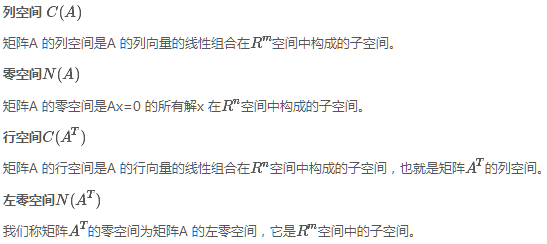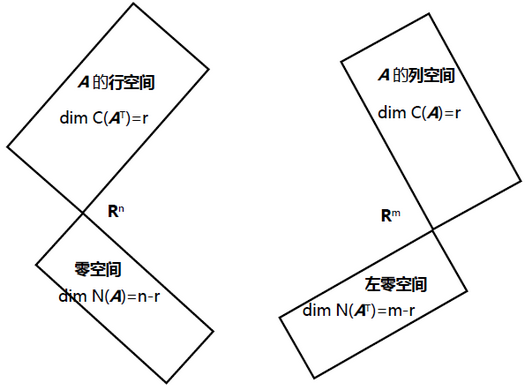接下来我们进行推导：

1) A$A$ 是m×n$m×n$ 的矩阵，在行空间中找个典型变量，记为v1$v_1$，然后变换到列空间的某向量，记为u1$u_1$，有u1=Av1$u_1=Av_1$。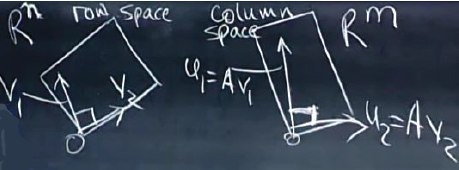那么这样的变换怎样合到一起，首先，这个行空间能找到一组正交基（格拉姆-施密特告诉我们以任意一组基开始，经过格拉姆-施密特正交化方法就可得到），但这组正交基经过A 变换后不一定能在列空间成为正交基，所以行空间中的正交基要找特殊的。考虑零空间，这些零空间体现在对角矩阵Σ$Σ$中是0$0$。

Av$Av$变换过程中，我希望转换得到的正交单位向量，所以u1,u2..$u_1,u_2..$是单位正交基，同时v1,v2..$v_1,v_2..$也是单位正交基，Av1$Av_1$ 等于u1$u_1$ 的一个倍数（可以理解为：在奇异值分解中，要找的是行空间的一组正交基，然后变换成列空间的一组正交基。现在要做的是，在A$A$的列空间中找到一组特殊的正交基v1,v2,⋯,vr$v_1,v_2,\cdots,v_r$，这组基在A$A$的作用下可以转换为A$A$的行空间中的一组正交基u1,u2,⋯,ur$u_1,u_2,\cdots,u_r$）。这种转换关系写成矩阵形式就是：

A[v1 v2 ⋯ vr]=[σ1u1 σ2u2 ⋯ σrur]=[u1 u2 ⋯ ur]⎡⎣⎢⎢⎢⎢⎢σ1σ2⋱σr⎤⎦⎥⎥⎥⎥⎥(1)A\Bigg[v_1\ v_2\ \cdots\ v_r\Bigg]=\Bigg[\sigma_1u_1\ \sigma_2u_2\ \cdots\ \sigma_ru_r\Bigg]=\Bigg[u_1\ u_2\ \cdots\ u_r\Bigg]\begin{bmatrix}\sigma_1& & & \\& \sigma_2& & \\& & \ddots& \\& & & \sigma_r\end{bmatrix}\tag{1}

即Av1=σ1u1, Av2=σ2u2,⋯,Avr=σrur$Av_1=\sigma_1u_1,\ Av_2=\sigma_2u_2,\cdots,Av_r=\sigma_ru_r$，这些σ$\sigma$是缩放因子，表示在转换过程中有拉伸或压缩。而A$A$的左零空间和零空间将体现在σ$\sigma$的零值中。

我们是想找：AV=UΣ$AV=UΣ$，（对于正定矩阵，这里是AQ=QΣ$AQ=QΣ$）但是发现与A维数不同$\color{red}{与A维数不同}$，我们要找到对任意A$A$都成立的一般形式。

2）如果A$A$ 存在零空间，那么行空间是r$r$ 维，零空间是n−r$n-r$ 维，我们同样可以取一组正交基。如果基零空间的向量为vr+1,...,vn$v_{r+1},...,v_n$，那么Avr+1$Av_{r+1}$ 将得到零向量，得到对角阵Σ对角线下方有一些0$0$。需要把整个Rn$R^n$ 空间的标准正交基完善成整个Rm$R^m$ 空间的标准正交基，在对角阵Σ中用0来完善，所以存在零空间时没问题，但行空间和列空间的基向量才是主要的$\color{red}{在对角阵Σ中用0 来完善，所以存在零空间时没问题，但行空间和列空间的基向量才是主要的}$。

因此算上左零、零空间，我们同样可以对左零、零空间取标准正交基，然后可以把(1)写为:

A[v1 v2 ⋯ vr vr+1 ⋯ vm]=[u1 u2 ⋯ ur ur+1 ⋯ un]⎡⎣⎢⎢⎢⎢⎢⎢σ1⋱σr⎤⎦⎥⎥⎥⎥⎥⎥(2)A\Bigg[v_1\ v_2\ \cdots\ v_r\ v_{r+1}\ \cdots\ v_m\Bigg]=\Bigg[u_1\ u_2\ \cdots\ u_r\ u_{r+1}\ \cdots \ u_n\Bigg]\left[\begin{array}{c c c|c}\sigma_1&&&\\&\ddots&&\\&&\sigma_r&\\\hline&&&\begin{bmatrix}0\end{bmatrix}\end{array}\right]\tag{2}

v1, ⋯, vr$v_1,\ \cdots,\ v_r$是行空间的标准正交基；
u1, ⋯, ur$u_1,\ \cdots,\ u_r$是列空间的标准正交基；
vr+1, ⋯, vn$v_{r+1},\ \cdots,\ v_n$是零空间的标准正交基；
ur+1, ⋯, um$u_{r+1},\ \cdots,\ u_m$是左零空间的标准正交基。

此时U是m×m正交矩阵，Σ是m×n对角矩阵，VT是n×n正交矩阵。$\color{red}{此时U是m\times m正交矩阵，\varSigma是m\times n对角矩阵，V^T是n\times n正交矩阵。}$

最终可以写为AV=UΣ$AV=U\varSigma$，可以看出这十分类似对角化的公式，矩阵A$A$被转化为对角矩阵Σ$\varSigma$，我们也注意到U, V$U,\ V$是两组不同的正交基。（在正定的情况下，U, V$U,\ V$都变成了Q$Q$。）。进一步可以写作A=UΣV−1$A=U\varSigma V^{-1}$，因为V$V$是标准正交矩阵所以可以写为A=UΣVT$A=U\varSigma V^T$

2.求解U和V$U和V$

找V$V$：ATA=VΣTUTUΣVT=VΣ2VT$A^TA=VΣ^TU^TUΣV^T=VΣ^2V^T$，得到的形式即：ATA=QΛQT$A^TA=QΛQ^T$，因此ATA$A^TA$ 是一个正定矩阵，它的特征向量标准正交组成Q$Q$，特征值是σ2$σ^2$组成Λ$Λ$。注意σ$σ$是Av=σu$Av=σu$的伸缩因子，σ2$σ^2$是ATA$A^TA$的特征值。σ$σ$取σ2$σ^2$ 的正平方根。
找U$U$：AAT=UΣVTVΣTUT=UΣ2UT$AA^T=UΣV^TVΣ^TU^T=UΣ^2U^T$，同样，形式即：AAT=QΛQT$AA^T =QΛQ^T$，因此AAT$AA^T$是一个正定矩阵，它的特征向量标准正交组成Q$Q$，特征值是σ2$σ^2$ 组成Λ$Λ$。注意σ$σ$是Av=σu$Av=σu$ 的伸缩因子，σ2$σ^2$
是AAT$AA^T$ 的特征值。

因此，AAT$AA^T$和ATA$A^TA$ 是特征值相同，特征向量不同的相似矩阵。

3.奇异值分解

奇异值分解的定义：
在线性代数的四个子空间中选出合适的基，v1$v_1$ 到vr$v_r$是行空间的标准正交基，用零空间的标准正交基vr+1$v_{r+1}$到vn$v_n$ 补充完整，u1$u_1$ 到ur$u_r$是列空间的标准正交基，用左零空间的标准正交基ur+1$u_{r+1}$ 到um$u_m$补充完整。A$A$ 乘以每一个v$v$ 对应一个u$u$的方向，Avi=σiui$Av_i=σ_iu_i$，可将矩阵对角化A=UΣV−1=UΣVT$A=UΣV^{-1}=UΣV^T$。

例子1：
A=[4−343]$A=\begin{bmatrix}4& 4\\-3& 3\end{bmatrix}$，我们需要找到：

行空间R2$\mathbb{R}^2$的标准正交基v1,v2$v_1,v_2$；
列空间R2$\mathbb{R}^2$的标准正交基u1,u2$u_1,u_2$；
σ1>0,σ2>0$\sigma_1>0, \sigma_2>0$。

在A=UΣVT$A=U\varSigma V^T$中有两个标准正交矩阵需要求解，我们希望一次只解一个，如何先将U$U$消去来求V$V$？
这个技巧会经常出现在长方形矩阵中：求ATA$A^TA$，这是一个对称正定矩阵（至少是半正定矩阵），于是有ATA=VΣTUTUΣVT$A^TA=V\varSigma^TU^TU\varSigma V^T$，由于U$U$是标准正交矩阵，所以UTU=I$U^TU=I$，而ΣTΣ$\varSigma^T\varSigma$是对角线元素为σ2$\sigma^2$的对角矩阵。
现在有ATA=V⎡⎣⎢⎢⎢⎢⎢σ1σ2⋱σn⎤⎦⎥⎥⎥⎥⎥VT$A^TA=V\begin{bmatrix}\sigma_1& & & \\& \sigma_2& & \\& & \ddots& \\& & & \sigma_n\end{bmatrix}V^T$，这个式子中V$V$即是ATA$A^TA$的特征向量矩阵而Σ2$\varSigma^2$是其特征值矩阵。

同理，我们只想求U$U$时，用AAT$AA^T$消掉V$V$即可。
我们来计算ATA=[44−33][4−343]=$A^TA=\begin{bmatrix}4& -3\\4& 3\end{bmatrix}\begin{bmatrix}4& 4\\-3& 3\end{bmatrix}=\begin{bmatrix}25& 7\\7& 25\end{bmatrix}$，对于简单的矩阵可以直接观察得到特征向量ATA=32, ATA[1−1]=18[1−1]$A^TA\begin{bmatrix}1\\1\end{bmatrix}=32\begin{bmatrix}1\\1\end{bmatrix},\ A^TA\begin{bmatrix}1\\-1\end{bmatrix}=18\begin{bmatrix}1\\-1\end{bmatrix}$，化为单位向量有σ1=32, v1=⎡⎣12√12√⎤⎦, σ2=18, v2=⎡⎣12√−12√⎤⎦$\sigma_1=32,\ v_1=\begin{bmatrix}\frac{1}{\sqrt{2}}\\\frac{1}{\sqrt{2}}\end{bmatrix},\ \sigma_2=18,\ v_2=\begin{bmatrix}\frac{1}{\sqrt{2}}\\-\frac{1}{\sqrt{2}}\end{bmatrix}$。

到目前为止，我们得到[4−343]=[u?u?u?u?][32−−√0018−−√]⎡⎣12√12√12√−12√⎤⎦$\begin{bmatrix}4& 4\\-3& 3\end{bmatrix}=\begin{bmatrix}u_?& u_?\\u_?& u_?\end{bmatrix}\begin{bmatrix}\sqrt{32}& 0\\0& \sqrt{18}\end{bmatrix}\begin{bmatrix}\frac{1}{\sqrt{2}}& \frac{1}{\sqrt{2}}\\\frac{1}{\sqrt{2}}& -\frac{1}{\sqrt{2}}\end{bmatrix}$。

接下来继续求解U$U$。
AAT=UΣVTVΣTUT=UΣ2UT$AA^T=U\varSigma V^TV\varSigma^TU^T=U\varSigma^2U^T$，求出AAT$AA^T$的特征向量即可得到